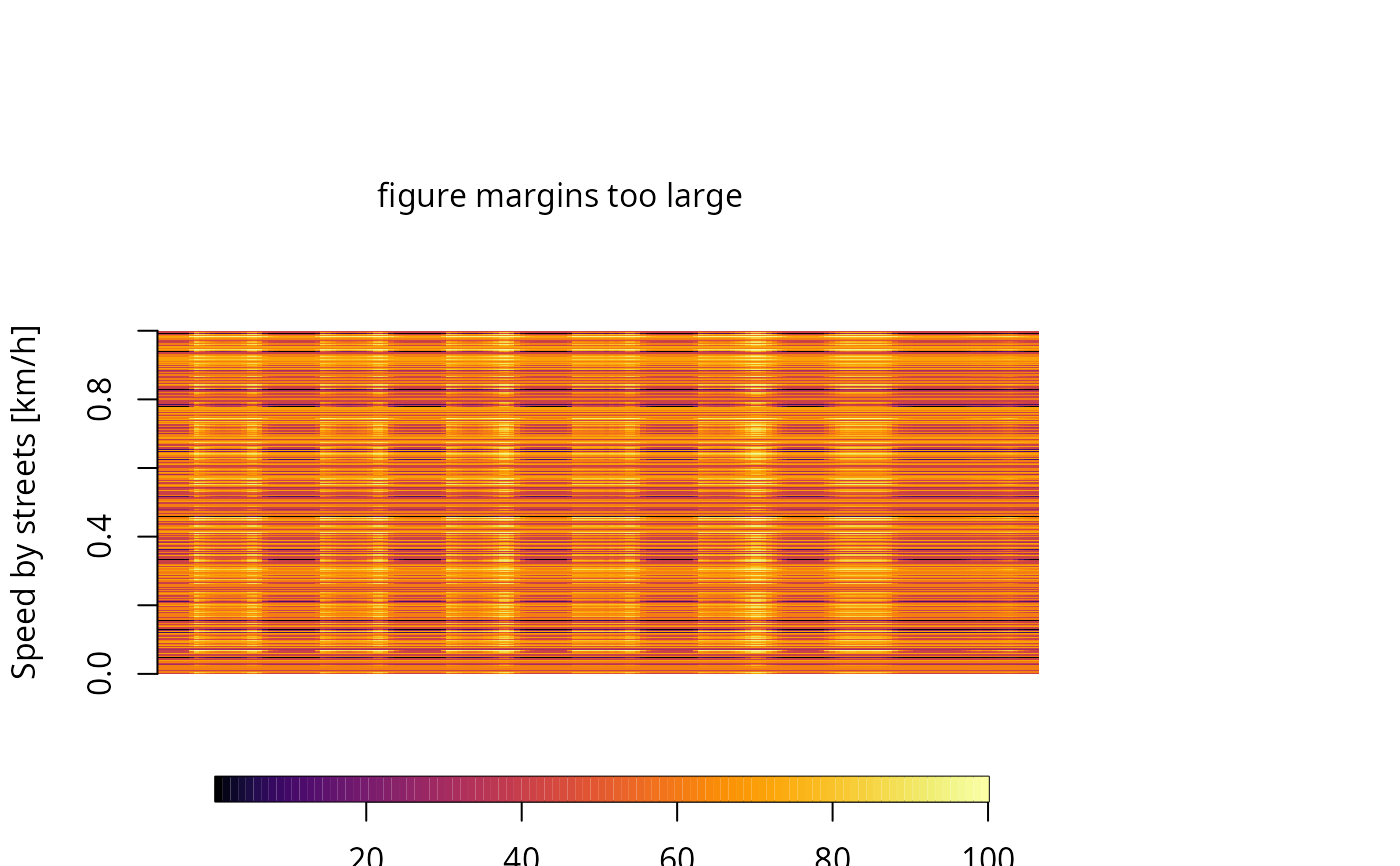netspeed Creates a dataframe of speeds for different hours and each link based on morning rush traffic data

netspeed(
q = 1,
ps,
ffs,
cap,
lkm,
alpha = 0.15,
beta = 4,
net,
scheme = FALSE,
dist = "km"
)

## Arguments

q

Data-frame of traffic flow to each hour (veh/h)

ps

Peak speed (km/h)

ffs

Free flow speed (km/h)

cap

lkm

alpha

Parameter of BPR curves

beta

Parameter of BPR curves

net

SpatialLinesDataFrame or Spatial Feature of "LINESTRING"

scheme

Logical to create a Speed data-frame with 24 hours and a default profile. It needs ffs and ps:

dist

String indicating the units of the resulting distance in speed. Default is units from peak speed ps

 00:00-06:00 ffs 06:00-07:00 average between ffs and ps 07:00-10:00 ps 10:00-17:00 average between ffs and ps 17:00-20:00 ps 20:00-22:00 average between ffs and ps 22:00-00:00 ffs

## Value

dataframe speeds with units or sf.

## Examples

{
data(net)
data(pc_profile)
pc_week <- temp_fact(net$ldv+net$hdv, pc_profile)
df <- netspeed(pc_week, net$ps, net$ffs, net$capacity, net$lkm, alpha = 1)
class(df)
plot(df) #plot of the average speed at each hour, +- sd
# net$ps <- units::set_units(net$ps, "miles/h")
# net$ffs <- units::set_units(net$ffs, "miles/h")
# df <- netspeed(pc_week, net$ps, net$ffs, net$capacity, net$lkm, alpha = 1)
# class(df)
# plot(df) #plot of the average speed at each hour, +- sd
# df <- netspeed(ps = net$ps, ffs = net$ffs, scheme = TRUE)
# class(df)
# plot(df) #plot of the average speed at each hour, +- sd
# dfsf <- netspeed(ps = net$ps, ffs = net$ffs, scheme = TRUE, net = net)
# class(dfsf)